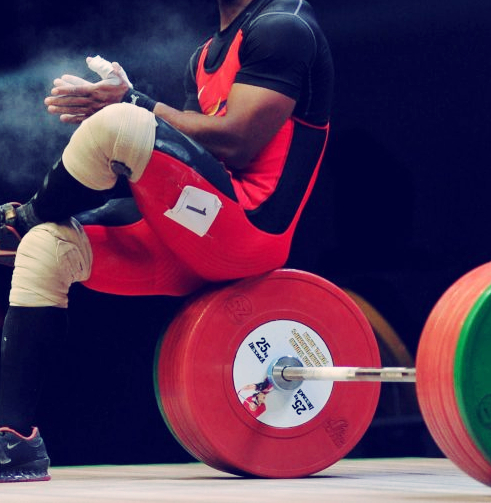Weightlifting

# Sinclair Coefficient

### The Sinclair Coefficient (2021-2024)

The Sinclair coefficients, derived statistically, are adjusted each Olympic year and are based on the Total World Records in the various bodyweight categories as of the previous several years.

The answer to the question “What would be the total of an athlete weighing x kg if he/she were an athlete in the heaviest class of the same level of ability?” is given by the formula: ACTUAL TOTAL x SINCLAIR COEFFICIENT = SINCLAIR TOTAL## Calculate yours

Male Female